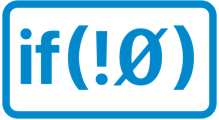# One feature that Swift is missing that Xojo has

ok… there may be more than one (although Swift still have more than Xojo)

But the one that I miss the most when moving from Xojo to Swift is ASSIGNS

``````Sub ChangeValue(a As Integer, b As Integer, Assigns c As Integer)
ChangeValue(5, 4) = 10
``````

There is NO EQUIVALENT in SwiftYou must use a syntax like this

``````func  ChangeValue(a : Int, b : Int,  c : Int)
ChangeValue(5, 4, 10)
``````

unless someone knows a trick that I haven’t discovered?

1 Like

In C++ the function can take two parameters and returns a reference to a property where you can write.
E.g. returns int&

## Xojo like properties with parameters in C++

Sorry Christian, none of that made a lick of sense.

And I’m no “C” programmer…cant define the += or = operator ?

its not exactly the same but maybe as close as you can get ?

The problem is that each variable would need a custom version of the operator, since different “logic” would need to be execute depending on what was being assigned etc… things easily accomplished with the Xojo “assigns”

that is more or less true with assigns as well
there’s no “write assigns once and it applies to each class etc” either
you kind of write one per type

the big difference I see is that theres no simple way to write multiple “assigns” per class IF you go the route of defining += + etc since assigns allows you to write several “assigns” methods

right … I could have

``````Sub ChangeValue(a As Integer, b As Integer, Assigns c As Integer)
call someotherfunction(a+b/c)
End Function

Sub foo(a As Integer, b As Integer, Assigns c As Integer)
call bar(c,b,a)
End Function
``````

if I created a custom operator, I would need a different class for each one, in which case I could simply use the SUBSCRIPT feature